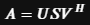1619766480

# Dimensionality reduction with Autoencoders versus PCA

## Introduction

Principal Component Analysis (PCA) is one of the most popular dimensionality reduction algorithms. PCA works by finding the axes that account for the larges amount of variance in the data which are orthogonal to each other. The iᵗʰ axis is called the iᵗʰ principal component (PC). The steps to perform PCA are:

• Standardize the data.
• Obtain the Eigenvectors and Eigenvalues from the covariance matrix or correlation matrix, or perform Singular Value Decomposition.

We will perform PCA with the implementation of sklearn: it uses Singular Value Decomposition (SVD) from scipy (scipy.linalg). SVD is a factorization of a 2D matrix A. it is written as:S is a 1D array which contains the singular values of AU and V^H are unitary (UU = UUᵗ = I). Most PCA implementation performs SVD to improve computational efficiency.

An Autoencoder (AE) on the other hand is a special kind of neural network which is trained to copy its input to its output. First, it maps the input to a latent space of reduced dimension, then code back the latent representation to the output. An AE learns to compress data by reducing the reconstruction error.

We will see in a moment how to implement and compare both PCA and Autoencoder results.

We will generate our data with make_classification from sklearn which will also give us some labels. We will use those labels to make comparisons between the efficiency of the clustering between our methods.

There will be three sections.

In the first we will implement a simple undercomplete linear autoencoder: that is, an autoencoder with a single layer which is of lower dimension than its input. The second section will introduce a Stacked Linear Autoencoder.

In the third section, we will try to modify the activation functions to address more complexity.

The results will be compared graphically with a PCA and in the end we will try to predict the classes using a simple random forest classification algorithm with cross validation.

#neural-networks #dimensionality-reduction #deep-learning #tensorflow #python